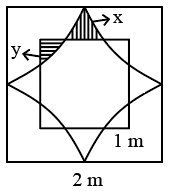Question 19

# A square of length 1 m is inside a square of length 2 m and four quarter circles are joined as shown in the figure. The value of y —x is given by,Solution

From the above figure area of  the region bounded by ABCDEFGH = Are of the square with side 2 - (4*quadrants with side 1cm + 4x)

=4-$$\pi\$$-4x

Which is same as the area of the square with side 1 cm -4y

4-$$\pi\$$-4x = 1-4y

4x-4y=3-$$\pi$$

y-x= $$\frac{\pi-3}{4}$$